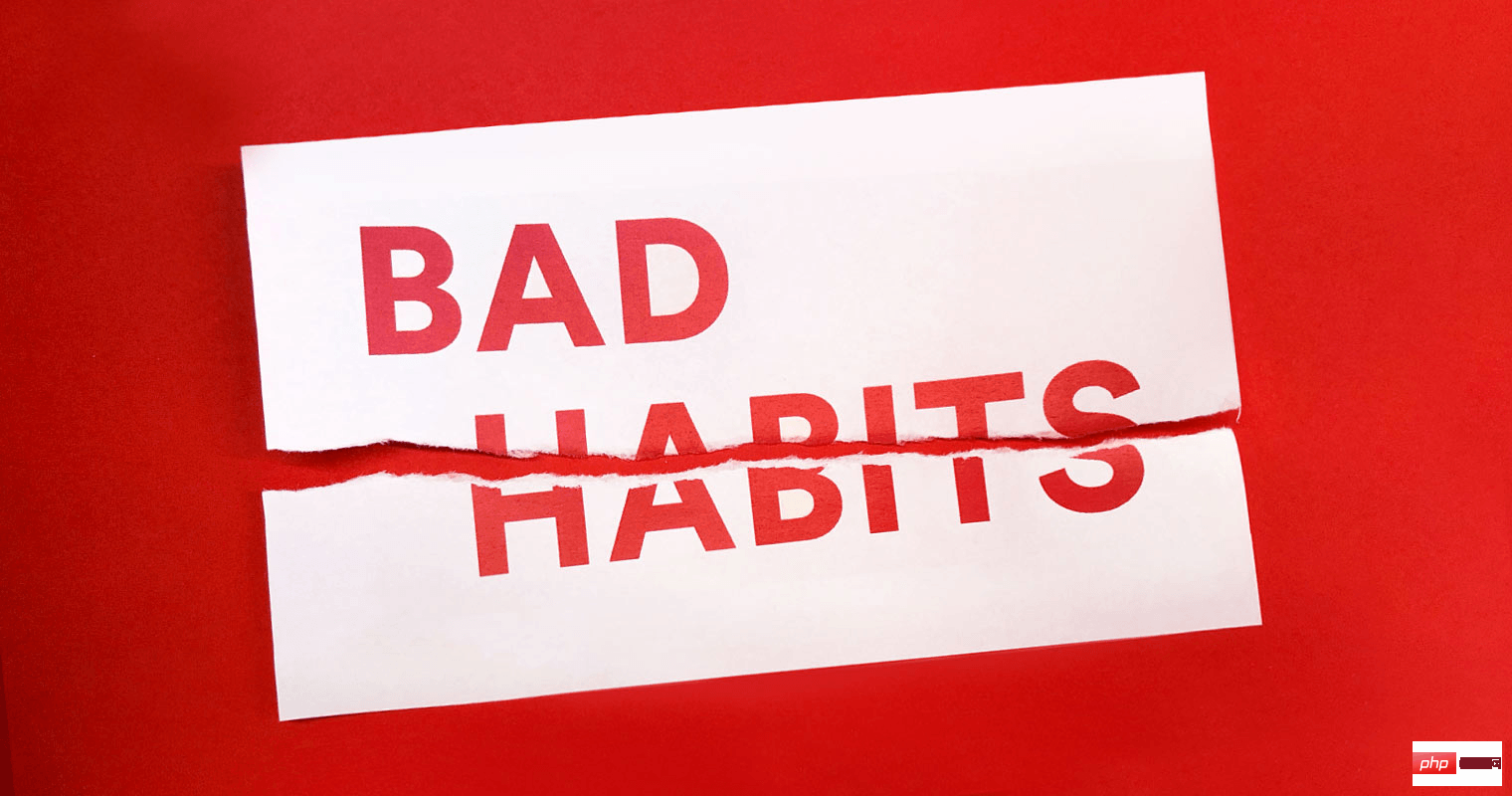# 你可能要纠正这5个PHP编码小陋习！_亿码酷站_亿码酷站

【亿码酷站-编程开发教程】收集全网优质教程及源码资源！```\$items = [];
// ...
if (count(\$items) > 0) {
foreach (\$items as \$item) {
// process on \$item ...
}
}```

`foreach` 以及数组函数 (`array_*`) 可以处理空数组。

• 不需要先进行测试
• 可减少一层缩进
```\$items = [];
// ...
foreach (\$items as \$item) {
// process on \$item ...
}```

```function foo(User \$user) {
if (!\$user->isDisabled()) {
// ...
// long process
// ...
}
}```

```function foo(User \$user) {
if (\$user->isDisabled()) {
return;
}

// ...
// 其他代码
// ...
}```

```\$a = null;
\$b = null;
\$c = null;
// ...

if (!isset(\$a) || !isset(\$b) || !isset(\$c)) {
throw new Exception("undefined variable");
}

// 或者

if (isset(\$a) && isset(\$b) && isset(\$c) {
// process with \$a, \$b et \$c
}

// 或者

\$items = [];
//...
if (isset(\$items['user']) && isset(\$items['user']['id']) {
// process with \$items['user']['id']
}```

```\$a = null;
\$b = null;
\$c = null;
// ...

if (!isset(\$a, \$b, \$c)) {
throw new Exception("undefined variable");
}

// 或者

if (isset(\$a, \$b, \$c)) {
// process with \$a, \$b et \$c
}

// 或者

\$items = [];
//...
if (isset(\$items['user'], \$items['user']['id'])) {
// process with \$items['user']['id']
}```

```\$name = "John Doe";
echo sprintf('Bonjour %s', \$name);```

```\$name = "John Doe";
printf('Bonjour %s', \$name);```

```\$items = [
'one_key' => 'John',
'search_key' => 'Jane',
];

if (in_array('search_key', array_keys(\$items))) {
// process
}```

```\$items = [
'one_key' => 'John',
'search_key' => 'Jane',
];

if (array_key_exists('search_key', \$items)) {
// process
}```

```if (isset(\$items['search_key'])) {
// process
}```

## linux php -v 命令找不到怎么解决

linux php -v 命令找不到怎么解决

php怎么判断是不是手机号

## php pdo扩展安装方法_编程技术_编程开发技术教程

php pdo扩展安装方法_编程技术_编程开发技术教程